• Slides: 20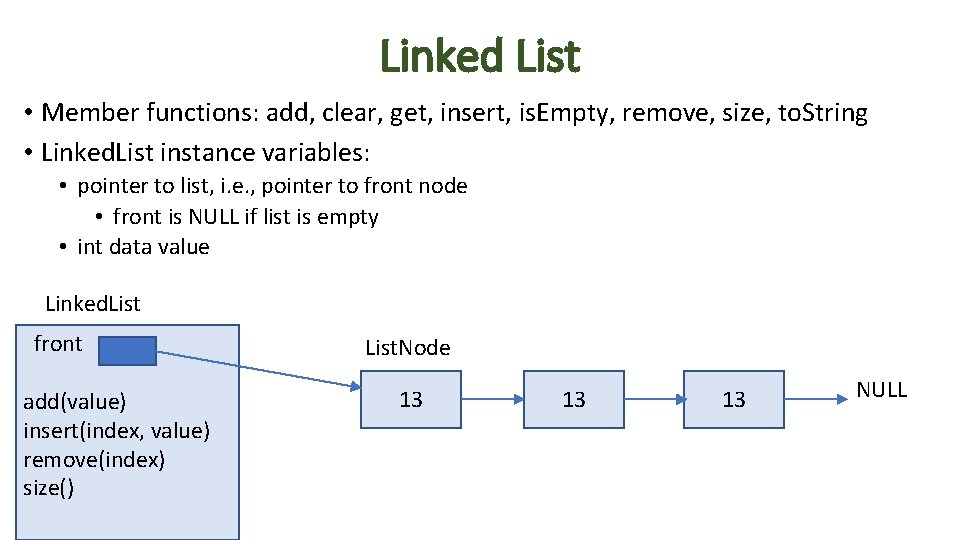Linked List • Member functions: add, clear, get, insert, is. Empty, remove, size, to. String • Linked. List instance variables: • pointer to list, i. e. , pointer to front node • front is NULL if list is empty • int data value Linked. List front add(value) insert(index, value) remove(index) size() List. Node 13 13 13 NULLList. Node struct (Linked. List. h) #ifndef _LINKEDLIST_H #define _LINKEDLIST_H struct List. Node { int data; List. Node* next; List. Node(int d = 0, List. Node* ptr = NULL) { data = d; next = ptr; } };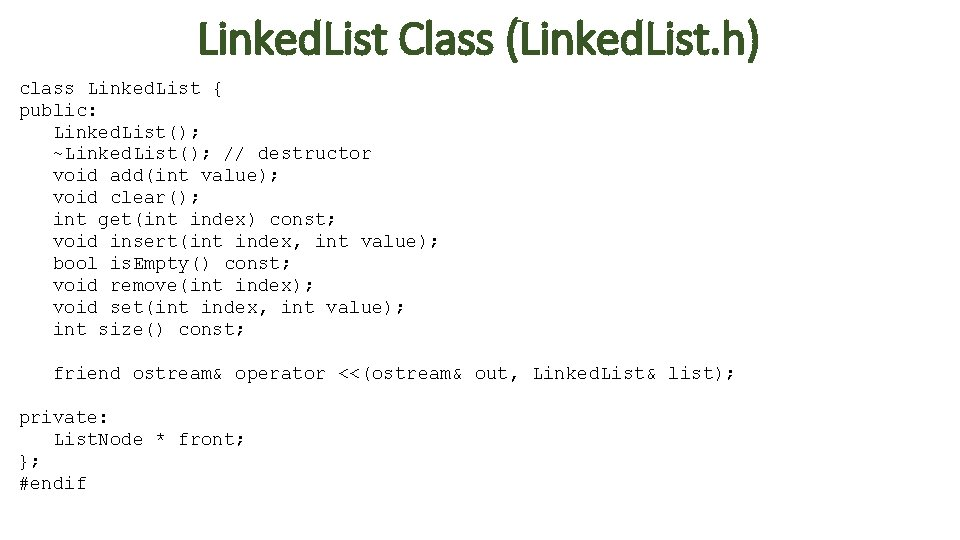Linked. List Class (Linked. List. h) class Linked. List { public: Linked. List(); ~Linked. List(); // destructor void add(int value); void clear(); int get(int index) const; void insert(int index, int value); bool is. Empty() const; void remove(int index); void set(int index, int value); int size() const; friend ostream& operator <<(ostream& out, Linked. List& list); private: List. Node * front; }; #endif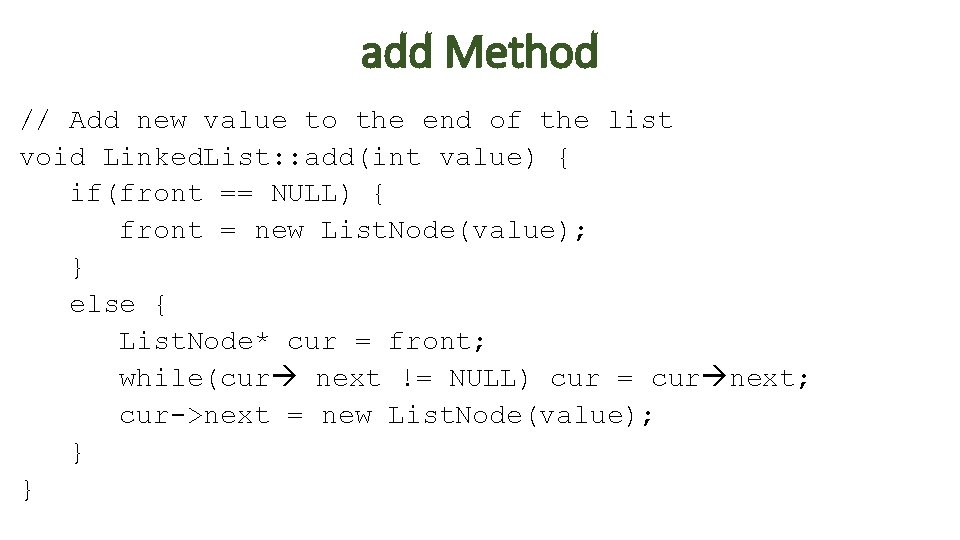add Method // Add new value to the end of the list void Linked. List: : add(int value) { if(front == NULL) { front = new List. Node(value); } else { List. Node* cur = front; while(cur next != NULL) cur = cur next; cur->next = new List. Node(value); } }get Method // Returns value at given index in list (first node at index 0). // Throws integer exception if specified index is not in the interval [0, size-1] int Linked. List: : get(int index) const { if(index < 0 || index >= size()) throw index; List. Node* cur = front; for(int i = 0; i < index; i++) cur = cur->next; return cur->data; } Exercise: // Sets value in ith node in the list to specified value. // Throws integer exception if specified index is not in interval [0, size-1]. void Linked. List: : set(int index, int value) {insert Method // Insert specified value at specified index in list. void Linked. List: : insert(int index, int value) { if(index < 0 || index >= size()) throw index; if(index == 0) { List. Node* list = front; front = new List. Node(value); front next = list; } else { List. Node* cur = front; for(int i = 0; i < index-1; i++) cur = cur next; // add new node after cur List. Node* new. Node = new List. Node(value); new. Node next = cur next; cur next = new. Node; } }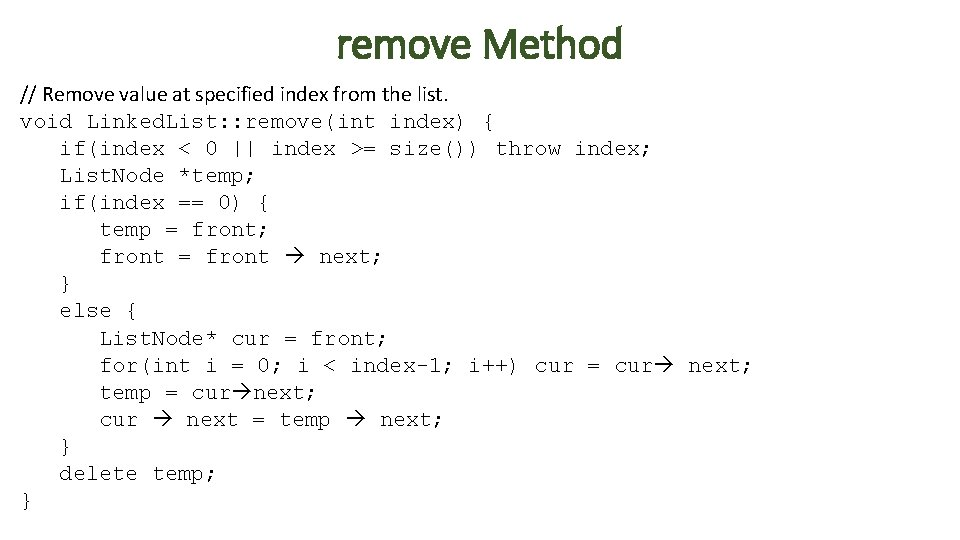remove Method // Remove value at specified index from the list. void Linked. List: : remove(int index) { if(index < 0 || index >= size()) throw index; List. Node *temp; if(index == 0) { temp = front; front = front next; } else { List. Node* cur = front; for(int i = 0; i < index-1; i++) cur = cur next; temp = cur next; cur next = temp next; } delete temp; }clear Method // Remove all values from the list. void Linked. List: : clear() { while(front != NULL) remove(0); }Destructor • Destructor should free all nodes in the list Linked. List: : ~Linked. List() { clear(); } • A default constructor is provided. But it will not delete the nodes in the linked list that front points to.Destructors, Copy Constructors & Copy Assignment Operators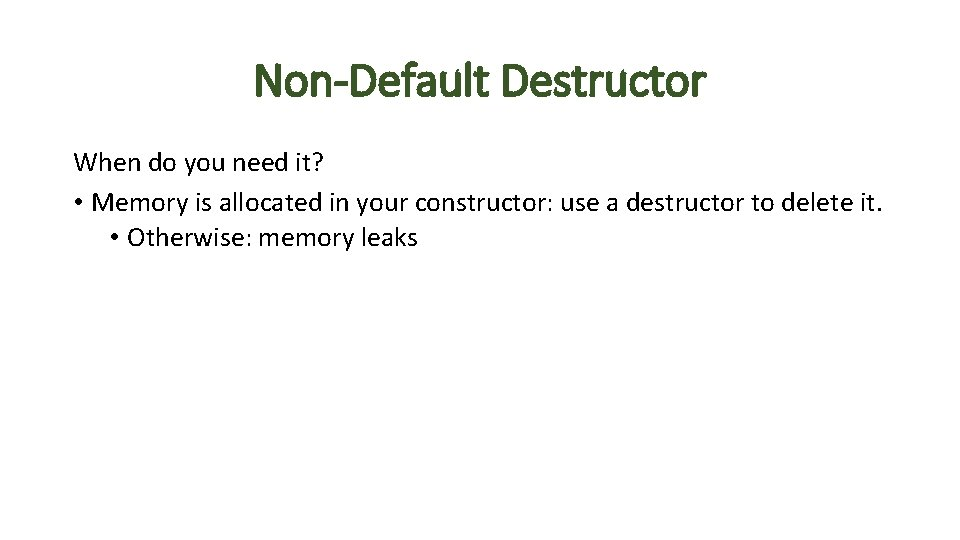Non-Default Destructor When do you need it? • Memory is allocated in your constructor: use a destructor to delete it. • Otherwise: memory leaksCopy Constructor & Copy Assignment Operator • Two ways to copy an object: • copy constructor: create a new object that's a copy of an existing object • copy assignment operator: set an existing object equal to another object • For existing Person object p: • Copy constructor: • Person p 2(p); // create new object p 2 using state of existing object p • Or equivalently: Person p 2 = p; • Copy assignment operator: • Person p 2 =. . . ; • p 2 = p; // p 2 is a Person object, and we want to set its state equal to that of p • If we don't define them in our classes, we get a default destructor, copy constructor and copy assignment operator. • When do we need to define our own? If an object has pointers or some other runtime allocation of a resource (e. g. , opening a file). • default copy constructor and assignment operator will do a shallow copy • default destructor will not deallocate memory allocated for an instance variable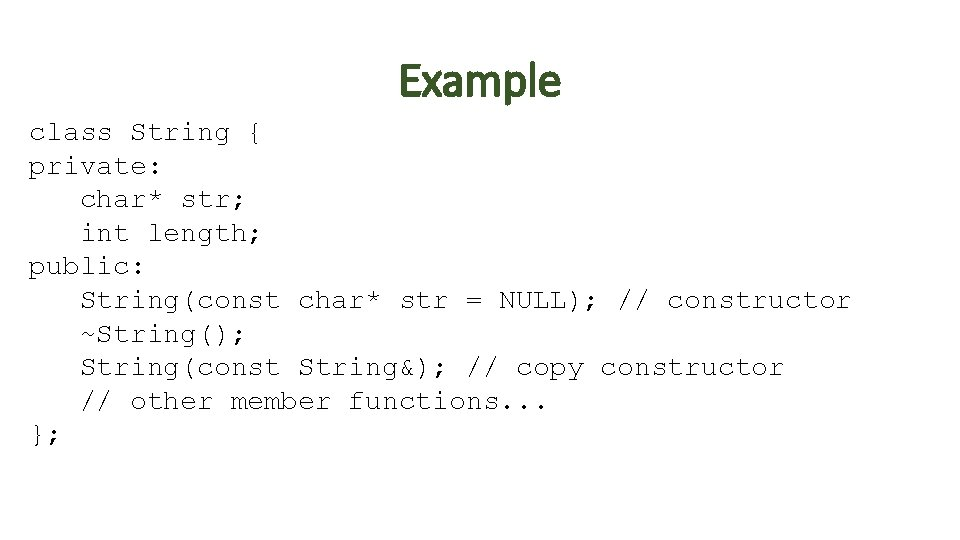Example class String { private: char* str; int length; public: String(const char* str = NULL); // constructor ~String(); String(const String&); // copy constructor // other member functions. . . };Shallow Copy • Default assignment operator and copy constructor produce a shallow copy String s 1("hello"); String s 2 = s 1; // default copy constructor invoked s 1 s 2 str length 5 'h' 'e' 'l' 5 'o' ''Default Destructor • Default destructor does not free the heap-allocated array that the str instance variable points to String* s 1 = new String("hello"); delete s 1; // default destructor results in memory leak s 1 str length 5 'h' 'e' 'l' 'o' ''Example String: : String(const char *s) { length = strlen(s); str = new char[length+1]; strcpy(str, s); } String: : ~String() { delete [] str; } // copy assignment operator String& String: : operator =(const String &s){ // copy constructor if(this != &s) { delete[] str; String: : String(const String& old. Str){ length = s. length; length = old. Str. length; str = new char[length]; str = new char[length+1]; strcpy(str, s. str); strcpy(str, old. Str. str); } } return *this; }Copy Constructor & Copy Assignment • To avoid implementing them, you can declare them to be private in your class. • Then if a client attempts to invoke them, they get a compile error. • The Rule of Three is a rule of thumb for C++: if your class needs to define any of copy constructor, assignment operator, destructor, then it needs all three of them.Exercise • Add copy constructor and copy assignment operator to Linked. List class